Skip to main content Accessibility help
Home
Hostname: page-component-564cf476b6-zvgck Total loading time: 0.194 Render date: 2021-06-21T07:56:15.127Z Has data issue: true Feature Flags: { "shouldUseShareProductTool": true, "shouldUseHypothesis": true, "isUnsiloEnabled": true, "metricsAbstractViews": false, "figures": true, "newCiteModal": false, "newCitedByModal": true, "newEcommerce": true }Forum of Mathematics, Sigma

# FORCING QUASIRANDOMNESS WITH TRIANGLES

Published online by Cambridge University Press:  02 April 2019

Corresponding
E-mail address:

## Abstract

We study forcing pairs for quasirandom graphs. Chung, Graham, and Wilson initiated the study of families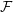${\mathcal{F}}$ of graphs with the property that if a large graph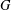$G$ has approximately homomorphism density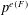$p^{e(F)}$ for some fixed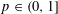$p\in (0,1]$ for every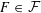$F\in {\mathcal{F}}$, then$G$ is quasirandom with density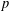$p$. Such families${\mathcal{F}}$ are said to be forcing. Several forcing families were found over the last three decades and characterizing all bipartite graphs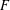$F$ such that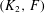$(K_{2},F)$ is a forcing pair is a well-known open problem in the area of quasirandom graphs, which is closely related to Sidorenko’s conjecture. In fact, most of the known forcing families involve bipartite graphs only.

We consider forcing pairs containing the triangle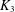$K_{3}$. In particular, we show that if$(K_{2},F)$ is a forcing pair, then so is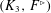$(K_{3},F^{\rhd })$, where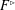$F^{\rhd }$ is obtained from$F$ by replacing every edge of$F$ by a triangle (each of which introduces a new vertex). For the proof we first show that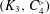$(K_{3},C_{4}^{\rhd })$ is a forcing pair, which strengthens related results of Simonovits and Sós and of Conlon et al.

## MSC classification

Type
Research Article
Information
Creative CommonsThis is an Open Access article, distributed under the terms of the Creative Commons Attribution licence (http://creativecommons.org/licenses/by/4.0/), which permits unrestricted re-use, distribution, and reproduction in any medium, provided the original work is properly cited.
Copyright
© The Author(s) 2019

## References

Aigner-Horev, E., Conlon, D., Hàn, H., Person, Y. and Schacht, M., ‘Quasirandomness in hypergraphs’, Electron. J. Combin. 25(3) (2018), Paper 3.34, 22 pages.Google Scholar
Alon, N., ‘Eigenvalues and expanders’, Combinatorica 6(2) (1986), 8396. Theory of Computing (Singer Island, Fla., 1984).CrossRefGoogle Scholar
Alon, N. and Chung, F. R. K., ‘Explicit construction of linear sized tolerant networks’, inProceedings of the First Japan Conference on Graph Theory and Applications (Hakone, 1986), Discrete Mathematics, 72 (Elsevier, North-Holland, 1988), 1519.CrossRefGoogle Scholar
Chung, F. R. K., ‘Quasi-random classes of hypergraphs’, Random Structures Algorithms 1(4) (1990), 363382.CrossRefGoogle Scholar
Chung, F. R. K., ‘Quasi-random hypergraphs revisited’, Random Structures Algorithms 40(1) (2012), 3948.CrossRefGoogle Scholar
Chung, F. R. K. and Graham, R. L., ‘Quasi-random hypergraphs’, Random Structures Algorithms 1(1) (1990), 105124.CrossRefGoogle Scholar
Chung, F. R. K. and Graham, R. L., ‘Quasi-random tournaments’, J. Graph Theory 15(2) (1991), 173198.CrossRefGoogle Scholar
Chung, F. R. K. and Graham, R. L., ‘Quasi-random set systems’, J. Amer. Math. Soc. 4(1) (1991), 151196.CrossRefGoogle Scholar
Chung, F. R. K. and Graham, R. L., ‘Maximum cuts and quasirandom graphs’, inRandom Graphs, vol. 2 (Poznań, 1989) (Wiley-Intersci. Publ., Wiley, New York, 1992), 2333.Google Scholar
Chung, F. R. K. and Graham, R. L., ‘Quasi-random subsets of Z n ’, J. Combin. Theory Ser. A 61(1) (1992), 6486.CrossRefGoogle Scholar
Chung, F. R. K. and Graham, R. L., ‘Sparse quasi-random graphs’, Combinatorica 22(2) (2002), 217244, Special issue: Paul Erdős and his mathematics.CrossRefGoogle Scholar
Chung, F. R. K., Graham, R. L. and Wilson, R. M., ‘Quasi-random graphs’, Combinatorica 9(4) (1989), 345362.CrossRefGoogle Scholar
Conlon, D., Fox, J. and Sudakov, B., ‘Hereditary quasirandomness without regularity’, Math. Proc. Cambridge Philos. Soc. 164(3) (2018), 385399.CrossRefGoogle Scholar
Conlon, D., Fox, J. and Sudakov, B., ‘An approximate version of Sidorenko’s conjecture’, Geom. Funct. Anal. 20(6) (2010), 13541366.CrossRefGoogle Scholar
Conlon, D., Fox, J. and Zhao, Y., ‘Extremal results in sparse pseudorandom graphs’, Adv. Math. 256 (2014), 206290.CrossRefGoogle Scholar
Conlon, D., Hàn, H., Person, Y. and Schacht, M., ‘Weak quasi-randomness for uniform hypergraphs’, Random Structures Algorithms 40(1) (2012), 138.CrossRefGoogle Scholar
Conlon, D., Kim, J. H., Lee, C. and Lee, J., ‘Some advances on Sidorenko’s conjecture’, J. Lond. Math. Soc. (2) 98(3) (2018), 593608.CrossRefGoogle Scholar
Conlon, D. and Lee, J., ‘Finite reflection groups and graph norms’, Adv. Math. 315 (2017), 130165.CrossRefGoogle Scholar
Cooper, J. N., ‘Quasirandom permutations’, J. Combin. Theory Ser. A 106(1) (2004), 123143.CrossRefGoogle Scholar
Frankl, P., Rödl, V. and Wilson, R. M., ‘The number of submatrices of a given type in a Hadamard matrix and related results’, J. Combin. Theory Ser. B 44(3) (1988), 317328.CrossRefGoogle Scholar
Frieze, A. and Kannan, R., ‘Quick approximation to matrices and applications’, Combinatorica 19(2) (1999), 175220.CrossRefGoogle Scholar
Gowers, W. T., ‘Quasirandom groups’, Combin. Probab. Comput. 17(3) (2008), 363387.CrossRefGoogle Scholar
Griffiths, S., ‘Quasi-random oriented graphs’, J. Graph Theory 74(2) (2013), 198209.CrossRefGoogle Scholar
Hàn, H., Person, Y. and Schacht, M., ‘Note on forcing pairs’, inThe Sixth European Conference on Combinatorics, Graph Theory and Applications (EuroComb 2011), Electronic Notes in Discrete Mathematics, 38 (Elsevier Science B. V., Amsterdam, 2011), 437442.Google Scholar
Hatami, H., ‘Graph norms and Sidorenko’s conjecture’, Israel J. Math. 175 (2010), 125150.CrossRefGoogle Scholar
Haviland, J. and Thomason, A., ‘Pseudo-random hypergraphs’, Discrete Math. 75(1–3) (1989), 255278, Graph Theory and Combinatorics (Cambridge, 1988).CrossRefGoogle Scholar
He, X., ‘Linear dependence between hereditary quasirandomness conditions’, Electron. J. Combin. 25(4) (2018), Paper 4.12, 14 pages.Google Scholar
Huang, H. and Lee, C., ‘Quasi-randomness of graph balanced cut properties’, Random Structures Algorithms 41(1) (2012), 124145.CrossRefGoogle Scholar
Hubai, T., Král, D., Parczyk, O. and Person, Y., ‘More non-bipartite forcing pairs’, submitted.Google Scholar
Janson, S. and Sós, V. T., ‘More on quasi-random graphs, subgraph counts and graph limits’, European J. Combin. 46 (2015), 134160.CrossRefGoogle Scholar
Kim, J. H., Lee, C. and Lee, J., ‘Two approaches to Sidorenko’s conjecture’, Trans. Amer. Math. Soc. 368(7) (2016), 50575074.CrossRefGoogle Scholar
Kohayakawa, Y. and Rödl, V., ‘Regular pairs in sparse random graphs. I’, Random Structures Algorithms 22(4) (2003), 359434.CrossRefGoogle Scholar
Kohayakawa, Y. and Rödl, V., ‘Szemerédi’s regularity lemma and quasi-randomness’, inRecent advances in algorithms and combinatorics, CMS Books Math./Ouvrages Math. SMC, 11 (Springer, New York, 2003), 289351.Google Scholar
Kohayakawa, Y., Rödl, V. and Skokan, J., ‘Hypergraphs, quasi-randomness, and conditions for regularity’, J. Combin. Theory Ser. A 97(2) (2002), 307352.CrossRefGoogle Scholar
Krivelevich, M. and Sudakov, B., ‘Pseudo-random graphs’, inMore Sets, Graphs and Numbers, Bolyai Society Mathematical Studies, 15 (Springer, Berlin, 2006), 199262.CrossRefGoogle Scholar
Lenz, J. and Mubayi, D., ‘Eigenvalues and linear quasirandom hypergraphs’, Forum Math. Sigma 3(e2) (2015), 26.CrossRefGoogle Scholar
Li, J. X. and Szegedy, B., ‘On the logarithmic calculus and Sidorenko’s conjecture’, Combinatorica to appear, Preprint, arXiv:1107.1153.Google Scholar
Lovász, L., Large Networks and Graph Limits, American Mathematical Society Colloquium Publications, 60 (American Mathematical Society, Providence, RI, 2012), xiv+475.CrossRefGoogle Scholar
Lovász, L. and Szegedy, B., ‘Szemerédi’s lemma for the analyst’, Geom. Funct. Anal. 17(1) (2007), 252270.CrossRefGoogle Scholar
Reiher, C. and Schacht, M., ‘Quasirandomness from hereditary subgraph densities’. Manuscript.Google Scholar
Rödl, V., ‘On universality of graphs with uniformly distributed edges’, Discrete Math. 59(1–2) (1986), 125134.CrossRefGoogle Scholar
Shapira, A., ‘Quasi-randomness and the distribution of copies of a fixed graph’, Combinatorica 28(6) (2008), 735745.CrossRefGoogle Scholar
Shapira, A. and Yuster, R., ‘The effect of induced subgraphs on quasi-randomness’, Random Structures Algorithms 36(1) (2010), 90109.CrossRefGoogle Scholar
Shapira, A. and Yuster, R., ‘The quasi-randomness of hypergraph cut properties’, Random Structures Algorithms 40(1) (2012), 105131.CrossRefGoogle Scholar
Sidorenko, A. F., ‘Inequalities for functionals generated by bipartite graphs’, Diskret. Mat. 3(3) (1991), 5065. (Russian); English transl., Discrete Math. Appl. 2(5) (1992) 489–504.Google Scholar
Simonovits, M., ‘Extremal graph problems, degenerate extremal problems, and supersaturated graphs’, inProgress in Graph Theory (Waterloo, Ont., 1982) (Academic Press, Toronto, ON, 1984), 419437.Google Scholar
Simonovits, M. and Sós, V. T., ‘Szemerédi’s partition and quasirandomness’, Random Structures Algorithms 2(1) (1991), 110.CrossRefGoogle Scholar
Simonovits, M. and Sós, V. T., ‘Hereditarily extended properties, quasi-random graphs and not necessarily induced subgraphs’, Combinatorica 17(4) (1997), 577596.CrossRefGoogle Scholar
Simonovits, M. and Sós, V. T., ‘Hereditary extended properties, quasi-random graphs and induced subgraphs’, Combin. Probab. Comput. 12(3) (2003), 319344, Combinatorics, Probability and Computing (Oberwolfach, 2001).CrossRefGoogle Scholar
Skokan, J. and Thoma, L., ‘Bipartite subgraphs and quasi-randomness’, Graphs Combin. 20(2) (2004), 255262.CrossRefGoogle Scholar
Szegedy, B., ‘An information theoretic approach to Sidorenko’s conjecture’, Preprint,arXiv:1406.6738.Google Scholar
Szemerédi, E., ‘Regular partitions of graphs’, inProblèmes combinatoires et théorie des graphes (Colloq. Internat. CNRS, Univ. Orsay, Orsay, 1976), Colloq. Internat. CNRS, 260 (CNRS, Paris, 1978), 399401.Google Scholar
Thomason, A., ‘Pseudorandom graphs’, inRandom Graphs ’85 (Poznań, 1985), North-Holland Mathematics Studies, 144 (North-Holland, Amsterdam, 1987), 307331.Google Scholar
Thomason, A., ‘Random graphs, strongly regular graphs and pseudorandom graphs’, inSurveys in Combinatorics 1987 (New Cross, 1987), London Mathematics Society Lecture Note Series, 123 (Cambridge University Press, Cambridge, 1987), 173195.Google Scholar
Towsner, H., ‘𝜎-algebras for quasirandom hypergraphs’, Random Structures Algorithms 50(1) (2017), 114139.CrossRefGoogle Scholar
Wilson, Richard M., ‘Cyclotomy and difference families in elementary abelian groups’, J. Number Theory 4 (1972), 1747.CrossRefGoogle Scholar
Yuster, Raphael, ‘Quasi-randomness is determined by the distribution of copies of a fixed graph in equicardinal large sets’, Combinatorica 30(2) (2010), 239246.CrossRefGoogle ScholarYou have AccessOpen access
1
Cited by

# Send article to Kindle

To send this article to your Kindle, first ensure no-reply@cambridge.org is added to your Approved Personal Document E-mail List under your Personal Document Settings on the Manage Your Content and Devices page of your Amazon account. Then enter the ‘name’ part of your Kindle email address below. Find out more about sending to your Kindle. Find out more about sending to your Kindle.

Note you can select to send to either the @free.kindle.com or @kindle.com variations. ‘@free.kindle.com’ emails are free but can only be sent to your device when it is connected to wi-fi. ‘@kindle.com’ emails can be delivered even when you are not connected to wi-fi, but note that service fees apply.

Find out more about the Kindle Personal Document Service.

FORCING QUASIRANDOMNESS WITH TRIANGLES
Available formats
×

# Send article to Dropbox

To send this article to your Dropbox account, please select one or more formats and confirm that you agree to abide by our usage policies. If this is the first time you use this feature, you will be asked to authorise Cambridge Core to connect with your <service> account. Find out more about sending content to Dropbox.

FORCING QUASIRANDOMNESS WITH TRIANGLES
Available formats
×

# Send article to Google Drive

To send this article to your Google Drive account, please select one or more formats and confirm that you agree to abide by our usage policies. If this is the first time you use this feature, you will be asked to authorise Cambridge Core to connect with your <service> account. Find out more about sending content to Google Drive.

FORCING QUASIRANDOMNESS WITH TRIANGLES
Available formats
×
×

#### Reply to:Submit a response

Please enter your response.

#### Your details

Please enter a valid email address.

#### Conflicting interests

Do you have any conflicting interests? *